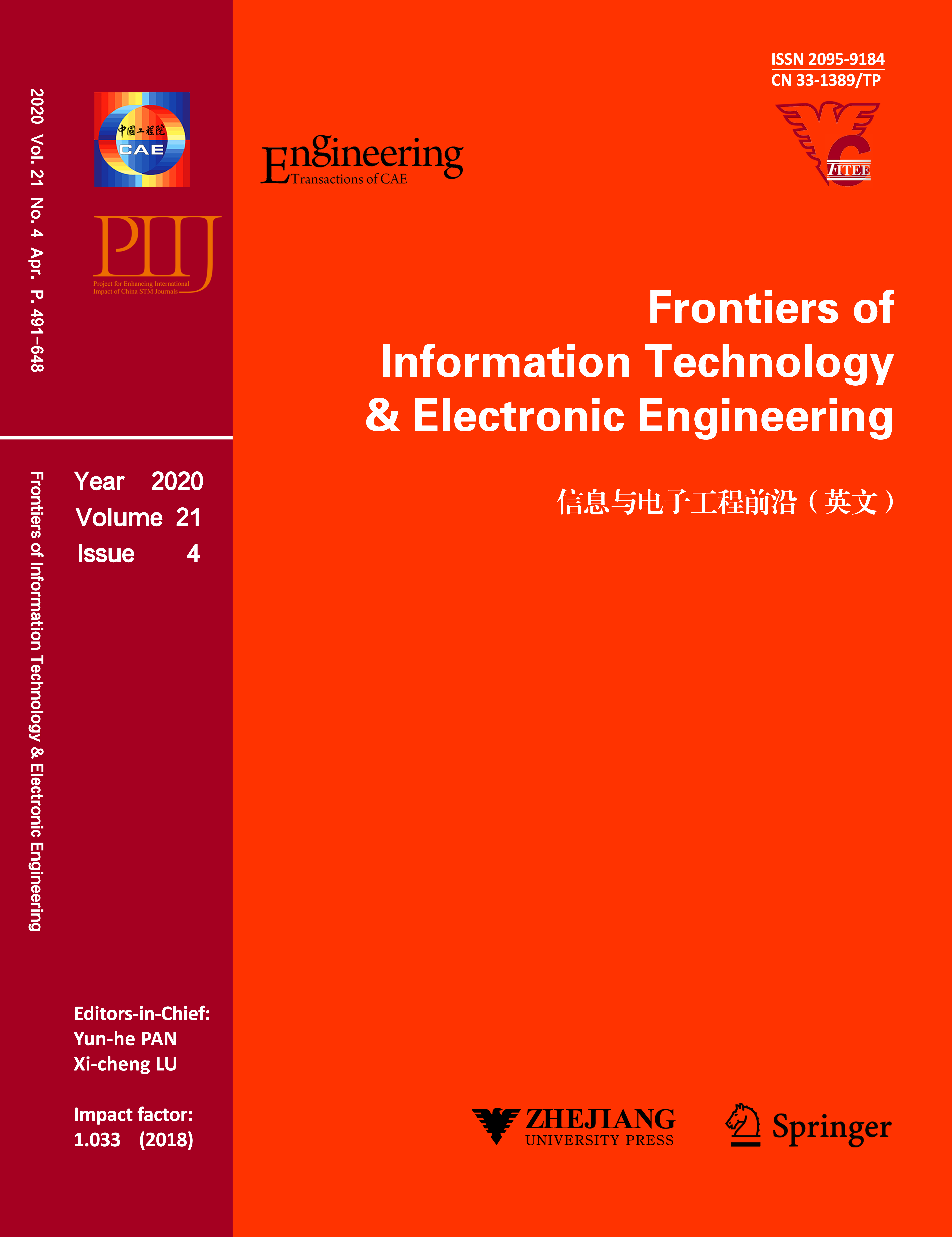,     et al.

,     et al.

,     et al.

,     et al.

,     et al.

,     et al.

,     et al.

,     et al.

,     et al.

,     et al.

,     et al.

,     et al.

,     et al.

,     et al.

,     et al.

,     et al.

,     et al.

,     et al.

,     et al.

,     et al.

,     et al.

,     et al.

,     et al.

#### Precursors and potential sources of ground-level ozone in suburban Shanghai

,     et al.#### Frontiers of Information Technology & Electronic Engineering

Volume 21,  Issue 4

,     et al.

,     et al.

,     et al.

,     et al.

,     et al.

,     et al.

,     et al.

,     et al.

,     et al.

,     et al.

,     et al.

,     et al.

,     et al.